## [C++]C++基础知识概述

article2023/9/29 5:21:33/

目录

C++基础知识概述：：

1.什么是C++

2.C++发展史

3.C++关键字

4.命名空间

5.C++的输入输出

6.缺省参数

7.函数重载

8.引用

9.内联函数

10.auto关键字(C++11)

11.基于范围的for循环(C++11)

12.指针空值—nullptr(C++11)

# C++基础知识概述：：

## 1.什么是C++

C语言是结构化和模块化的语言，适合处理较小规模的程序。对于复杂的问题，规模较大的程序，需要高度的抽象和建模时，C语言则不合适，为了解决软件危机，20世纪80年代，计算机界提出了OOP(object oriented programming：面向对象)思想，支持面向对象的程序设计语言应运而生。

1982年，Bjarne Stroustrup博士在C语言的基础上引入并补充了面向对象的概念，发明了一种新的程序语言，为了表达该语言与C语言的渊源关系，命名为C++，因此，C++是基于C语言而产生的，它既可以进行C语言的过程化程序设计，又可以进行以抽象数据类型为特点的基于对象的程序设计，还可以进行面向对象的程序设计。

## 2.C++发展史

1979年，贝尔实验室的本贾尼等人试图分析unix内核的时候，试图将内核模块化，于是在C语言的基础上进行了扩展，增加了类的机制，完成了一个可以运行的预处理程序，称之为C with classes语言的发展就像是练功打怪升级一样，也是逐步递进，由浅入深的过程，下面是C++的历程版本：

 阶段 内容 C with classes 类及派生类、公有和私有成员、类的构造和析构、友元、内联函数、赋值运算符、重载等 C++1.0 添加虚函数概念，函数和运算符重载，引用、常量等 C++2.0 更加完善支持面向对象，新增保护成员、多重继承、对象的初始化、抽象类、静态成员以及const 成员函数 C++3.0 进一步完善，引入模板，解决多重继承产生的二义性问题和相应构造和析构的处理 C++98 C++ 标准第一个版本 ，绝大多数编译器都支持，得到了国际标准化组织 (ISO) 和美国标准化协会认可，以模板方式重写 C++ 标准库，引入了 STL( 标准模板库 ) C++03 C++ 标准第二个版本，语言特性无大改变，主要：修订错误、减少多异性 C++05 C++ 标准委员会发布了一份计数报告 (Technical Report ， TR1) ，正式更名C++0x，即：计划在本世纪第一个 10 年的某个时间发布 C++11 增加了许多特性，使得 C++ 更像一种新语言，比如：正则表达式、基于范围 for 循环、auto 关键字、新容器、列表初始化、标准线程库等 C++14 对 C++11 的扩展，主要是修复 C++11 中漏洞以及改进，比如：泛型的 lambda 表达式，auto 的返回值类型推导，二进制字面常量等 C++17 在 C++11 上做了一些小幅改进，增加了 19 个新特性，比如： static_assert() 的文本信息可选，Fold 表达式用于可变的模板， if 和 switch 语句中的初始化器等 C++20 自 C++11 以来最大的发行版 ，引入了许多新的特性，比如： 模块 (Modules) 、协 程 (Coroutines) 、范围 (Ranges) 、概念 (Constraints) 等重大特性，还有对已有特性的更新：比如Lambda 支持模板、范围 for 支持初始化等 C++23 制定中

## 3.C++关键字

C++总计63个关键字，C语言32个关键字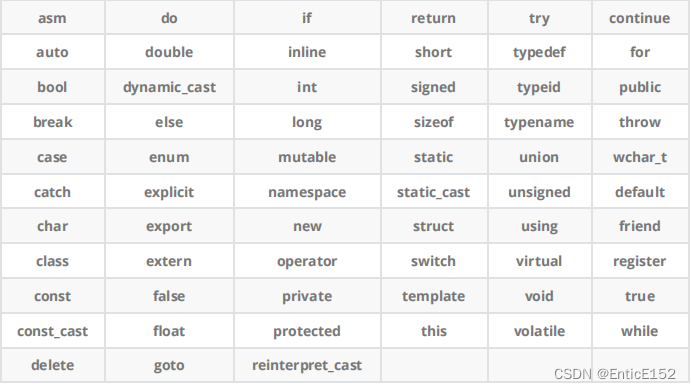## 4.命名空间

``````#include <stdio.h>
#include <stdlib.h>
int rand = 10;
// C语言没办法解决类似这样的命名冲突问题，所以C++提出了namespace来解决
int main()
{
printf("%d\n", rand);
return 0;
}
// 编译后后报错：error C2365: “rand”: 重定义；以前的定义是“函数”``````

``````//1.正常的命名空间定义
namespace bit
{
// 命名空间中可以定义变量/函数/类型
int rand = 10;
{
return left + right;
}
//2. 命名空间可以嵌套
// test.cpp
namespace N1
{
int a;
int b;
{
return left + right;
}
namespace N2
{
int c;
int d;
int Sub(int left, int right)
{
return left - right;
}
}
}
//3.同一个工程中允许存在多个相同名称的命名空间,编译器最后会合成同一个命名空间中。
// ps：一个工程中的test.h和上面test.cpp中两个N1会被合并成一个
// test.h
namespace N1
{
int Mul(int left, int right)
{
return left * right;
}
}``````

``````namespace bit
{
// 命名空间中可以定义变量/函数/类型
int a = 0;
int b = 1;
{
return left + right;
}
struct Node
{
struct Node* next;
int val;
};
}
int main()
{
// 编译报错：error C2065: “a”: 未声明的标识符
printf("%d\n", a);
return 0;
}
``````

1.加命名空间名称及作用域限定符
``````int main()
{
printf("%d\n", N::a);
return 0;
}``````
2.使用using将命名空间中某个成员引入
``````using N::b;
int main()
{
printf("%d\n", N::a);
printf("%d\n", b);
return 0;
}``````
3.使用using namespace 命名空间名称引入
``````using namespce N;
int main()
{
printf("%d\n", a);
printf("%d\n", b);
return 0;
}``````

``````#include<iostream>
int main()
{
//流插入
//自动识别类型
std::cout << "hello world" << std::endl;
int i = 10;
double j = 10.11;
std::cout << i << " " << j << std::endl;
return 0;
}
#include<stdio.h>
int a = 10;
int main()
{
int a = 1;
printf("%d\n", a);
//::域作用限定符 ::的左边是空白默认代表全局域
printf("%d\n", ::a);
return 0;
}
//命名空间中的变量是全局变量，定义在函数中的变量是局部变量，存在栈上，函数调用会建立栈帧，出作用域即被销毁
//命名空间不影响变量的生命周期，只是一个限定域，影响的是编译器的查找规则
//默认查找规则：先在局部找，再全局找
//命名空间的作用：避免命名冲突
//命名空间嵌套使用时定义的变量均为全局变量，只是调用方式要多嵌套一层命名空间
//同一个工程中允许存在多个相同名称的命名空间，编译器最后会合成同一个命名空间中
#include<iostream>
//using namespace std;
//用起来方便，命名空间的隔离墙拆了
//日常练习 小程序这么用可以，项目最好避免这么用
int cout = 0;
int main()
{
cout << "hello world" << endl;
return 0;
}
//报错:cout命名冲突
#include <iostream>
//指定展开—常用展开，自己定义的时候避免跟常用重名即可
//using std::cout;
//int main()
//{
//	cout << "hello world" << std::endl;
//	return 0;
//}``````

## 5.C++的输入输出

C++中的"hello world"

``````#include<iostream>
// std是C++标准库的命名空间名，C++将标准库的定义实现都放到这个命名空间中
using namespace std;
int main()
{
cout<<"hello world!!!"<<endl;
return 0;
}``````

1. 使用 cout 标准输出对象 ( 控制台 ) cin 标准输入对象 ( 键盘 ) 时，必须 包含 < iostream > 头文件

2. cout cin 是全局的流对象， endl 是特殊的 C++ 符号，表示换行输出，他们都包含在包含 <
iostream > 头文件中。
3. << 是流插入运算符， >> 是流提取运算符
4. 使用 C++ 输入输出更方便，不需要像 printf/scanf 输入输出时那样，需要手动控制格式，C++的输入输出可以自动识别变量类型。
5. 实际上 cout cin 分别是 ostream istream 类型的对象。
``````#include<iostream>
using namespace std;
int main()
{
//可以自动识别变量的类型 相比C的优势 printf/scanf
// << 流插入运算符
cout << "hello world" << endl;
int a;
double b;
char c;
// >> 流提取运算符
cin >> a;
cin >> b >> c;
return 0;
}``````

std命名空间的使用习惯：

1. 在日常练习中，建议直接 using namespace std 即可，这样就很方便。
2. using namespace std 展开，标准库就全部暴露出来了，如果我们定义跟库重名的类型 / 对象/ 函数，就存在冲突问题。该问题在日常练习中很少出现，但是项目开发中代码较多、规模大，就很容易出现。所以建议在项目开发中使用，像std::cout 这样使用时指定命名空间 + using std::cout展开常用的库对象 / 类型等方式。

## 6.缺省参数

``````void Func(int a = 0)
{
cout<<a<<endl;
}
int main()
{
Func();     // 没有传参时，使用参数的默认值
Func(10);   // 传参时，使用指定的实参
return 0;
}``````

1.全缺省参数

``````void Func(int a = 10, int b = 20, int c = 30)
{
cout<<"a = "<<a<<endl;
cout<<"b = "<<b<<endl;
cout<<"c = "<<c<<endl;
}``````

2.半缺省参数

``````void Func(int a, int b = 10, int c = 20)
{
cout<<"a = "<<a<<endl;
cout<<"b = "<<b<<endl;
cout<<"c = "<<c<<endl;
}``````

1.半缺省参数必须从右往左依次来给出，不能间隔着给。

2.缺省参数不能在函数声明和定义中同时出现，声明定义分离时，规定在声明中给出缺省值。

``````  //a.h
void Func(int a = 10);
// a.cpp
void Func(int a = 20)
// 注意：如果生命与定义位置同时出现，恰巧两个位置提供的值不同，那编译器就无法确定到底该

3.缺省值必须是常量或者全局变量。

4.C语言不支持。

## 7.函数重载

``````#include<iostream>
using namespace std;
//1、参数类型不同
{
cout << "int Add(int left, int right)" << endl;
return left + right;
}
{
cout << "double Add(double left, double right)" << endl;
return left + right;
}
//2、参数个数不同
void f()
{
cout << "f()" << endl;
}
void f(int a)
{
cout << "f(int a)" << endl;
}
//3、参数类型顺序不同
void f(int a, char b)
{
cout << "f(int a,char b)" << endl;
}
void f(char b, int a)
{
cout << "f(char b, int a)" << endl;
}
int main()
{
f();
f(10);
f(10, 'a');
f('a', 10);
return 0;
}``````

C++支持函数重载的原理——名字修饰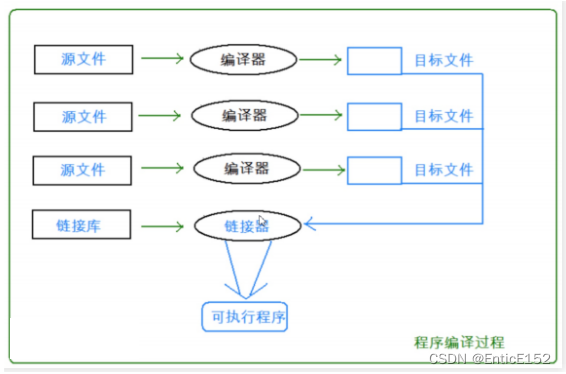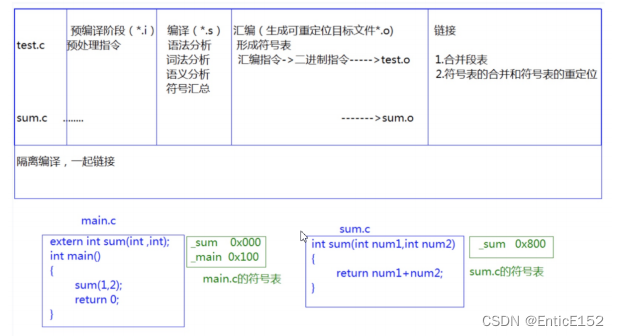4.由于Windows下VS的修饰规则过于复杂，而Linux下g++的修饰规则简单易懂，下面我们使用了g++演示了这个修饰后的名字。

5.通过下面我们可以看出gcc的函数修饰后名字不变，而g++的函数修饰后变成Z+函数长度+函数名+类型首字母。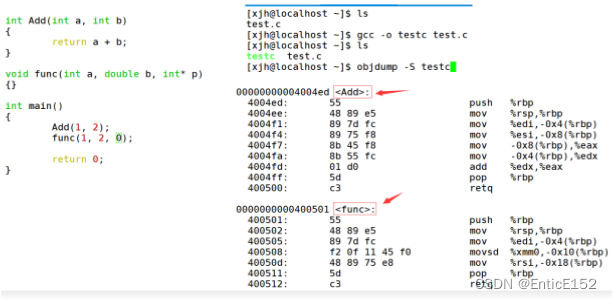采用C++编译器编译后结果：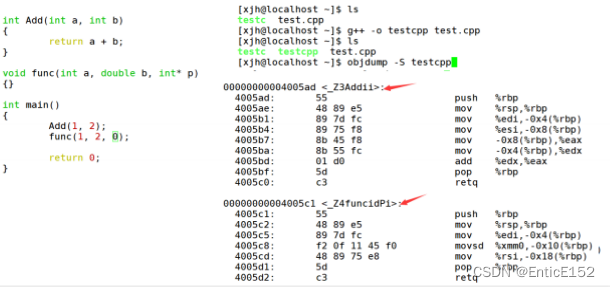Window下名字修饰规则：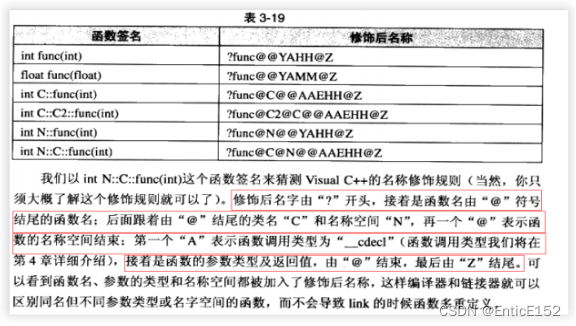6.通过这里就理解了C语言没办法支持重载，因为同名函数没办法区分，而C++是通过函数修饰规则来区分，只要参数不同，修饰出来的名字就不一样，就支持了重载。

7.如果两个函数名和参数是一样的，返回值不同是不构成重载的，因为调用时编译器没办法区分，避免调用的二义性。

## 8.引用

``````int& Count()
{
int n = 0;
n++;
return n;
}
void Func()
{
int x = 100;
}
int main()
{
int& ret = Count();
cout << ret << endl;
cout << ret << endl;
Func();
cout << ret << endl;
}
//三次ret的结果为1 随机值 100
//cout本身是一次函数调用，第一次cout，ret作为参数传递，第二次的n则被覆盖
//结论:出了函数作用域，返回变量不存在了，不能用引用返回，因为引用返回的结果是未定义的
//出了函数作用域，返回变量存在才能用引用返回
//正确玩法:
int Count1()
{
int n = 0;
n++;
return n;
}
int& Count2()
{
static int n = 0;
n++;
return n;
}
int main()
{
int ret1 = Count1();
int& ret2 = Count2();
cout << ret1 << endl;
cout << ret2 << endl;
return 0;
}``````

``````void TestRef()
{
int a = 10;
int& ra = a;
printf("%p\n", &a);
printf("%p\n", &ra);
}``````

1.引用在定义时必须初始化

2.一个变量可以有多个引用

3.引用一旦引用一个实体，再不能引用其他实体

``````void TestRef()
{
int a = 10;
//int& ra;//该条语句编译时会出错
int& ra = a;
int& rra = a;
printf("%p %p %p\n", &a, &ra, &rra);
}
``````

``````int main()
{
int a = 0;
//权限平移
int& ra = a;
//指针和引用赋值中 权限可以缩小 但是不能放大
const int b = 1;
//我引用你 权限放大 不行
//int& rb = b;
//我引用你 我的权限缩小了 可以
const int& rra = a;
//rra++ const修饰 不能++
a++;
return 0;
}
//一般用引用做参数都是用const引用
//类型转换的本质是中间产生了临时变量
//临时变量具有常性不可修改
int main()
{
const int& b = 10;
double d = 10;
cout << (int)d << endl;
int i = (int)d;//可以
//int& ri = d;//不可以
//ri是d的临时变量的别名 所以ri的值为12
const int& ri = d;//可以
cout << ri << endl;
return 0;
}
//传值返回的函数不能用引用接收 要用const引用
//语法上面 ra是a的别名 不开空间
//底层实现 引用是使用指针实现的``````

1.做参数

``````void Swap(int& left, int& right)
{
int temp = left;
left = right;
right = temp;
}``````

1.减少拷贝，提高效率
2.作输出型参数，函数中修改形参，实参也修改

2.做返回值

``````int& Count()
{
static int n = 0;
n++;
// ...
return n;
}``````

1.减少拷贝，提高效率
2.修改返回值

``````int& Add(int a, int b)
{
int c = a + b;
return c;
}
int main()
{
cout << "Add(1, 2) is :"<< ret <<endl;
return 0;
}``````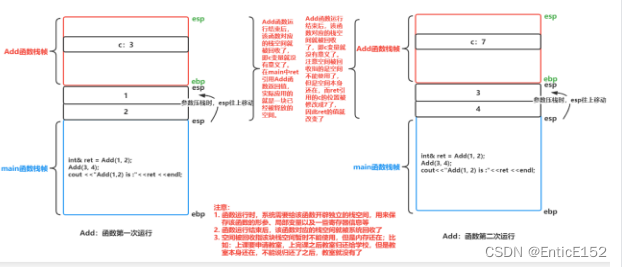``````#include <time.h>
struct A{ int a; };
void TestFunc1(A a){}
void TestFunc2(A& a){}
void TestRefAndValue()
{
A a;
// 以值作为函数参数
size_t begin1 = clock();
for (size_t i = 0; i < 10000; ++i)
TestFunc1(a);
size_t end1 = clock();
// 以引用作为函数参数
size_t begin2 = clock();
for (size_t i = 0; i < 10000; ++i)
TestFunc2(a);
size_t end2 = clock();
// 分别计算两个函数运行结束后的时间
cout << "TestFunc1(A)-time:" << end1 - begin1 << endl;
cout << "TestFunc2(A&)-time:" << end2 - begin2 << endl;
}``````

``````#include <time.h>
struct A
{
int a;
};
A a;
// 值返回
A TestFunc1() { return a;}
// 引用返回
A& TestFunc2(){ return a;}
void TestReturnByRefOrValue()
{
// 以值作为函数的返回值类型
size_t begin1 = clock();
for (size_t i = 0; i < 100000; ++i)
TestFunc1();
size_t end1 = clock();
// 以引用作为函数的返回值类型
size_t begin2 = clock();
for (size_t i = 0; i < 100000; ++i)
TestFunc2();
size_t end2 = clock();
// 计算两个函数运算完成之后的时间
cout << "TestFunc1 time:" << end1 - begin1 << endl;
cout << "TestFunc2 time:" << end2 - begin2 << endl;
}``````

``````int main()
{
int a = 10;
int& ra = a;
cout<<"&a = "<<&a<<endl;
cout<<"&ra = "<<&ra<<endl;
return 0;
}``````

``````int main()
{
int a = 10;
int& ra = a;
ra = 20;
int* pa = &a;
*pa = 20;
return 0;
}``````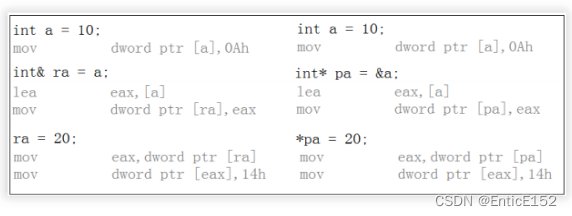引用和指针的不同点：

1.引用概念上定义一个变量的别名，指针存储一个变量地址。

2.引用在定义时必须初始化，指针没有要求。

3.引用在初始化时引用一个实体后，就不能再引用其他实体，而指针可以在任何时候指向任何一个同类型实体。

4.没有NULL引用，但有NULL指针。

5.在sizeof中含义不同，引用结果为引用类型的大小，但指针始终是地址空间所占字节个数(32位平台下4byte)。

6.引用自加即引用的实体增加1，指针自加即指针向后偏移一个类型的大小。

7.有多级指针但是没有多级引用。

8.引用比指针使用起来相对安全。

## 12.指针空值—nullptr(C++11)

### React中使用lodash防抖失效解决

React中使用lodash防抖失效解决 import {Input} from antd; import lodash from lodash; // lodash下的防抖函数 const debounce lodash.debounce; // 防抖打印&#xff0c;希望输入的时候&#xff0c;延迟0.5s后打印值 const getSuggestion debounce((val:string) > {co…

### SpringCloud微服务技术栈.黑马跟学(九)

SpringCloud微服务技术栈.黑马跟学 九今日目标1.分布式事务问题1.1.本地事务1.2.分布式事务1.3.演示分布式事务问题2.理论基础2.1.CAP定理2.1.1.一致性2.1.2.可用性2.1.3.分区容错2.1.4.矛盾2.2.BASE理论2.3.解决分布式事务的思路3.初识Seata3.1.Seata的架构3.2.部署TC服务一、…

### 什么是RabbitMQ？有什么用如何使用？一文回答

RabbitMQ RabbitMQ channel&#xff1a;操作MQ的工具exchange&#xff1a;交换机&#xff0c;路由消息到队列中queue&#xff1a;队列&#xff0c;缓存消息virtual host&#xff1a;虚拟主机&#xff0c;对queue&#xff0c;exchange等资源的逻辑分组 MQ模型 基本消息队列工作…

### Java 8 - Lambda 表达式

1. 函数式接口 当一个接口中只有一个非 default 修饰的方法&#xff0c;这个接口就是一个函数式接口用 FunctionalInterface 标注 1&#xff09;只有一个抽象方法 FunctionalInterface public interface MyInterface {void print(int x); } 2&#xff09;只有一个抽象方法和…